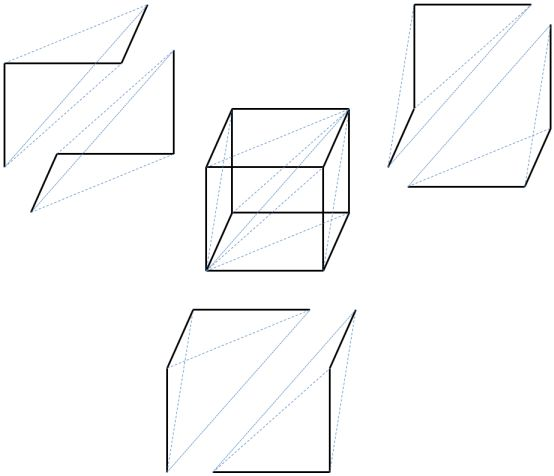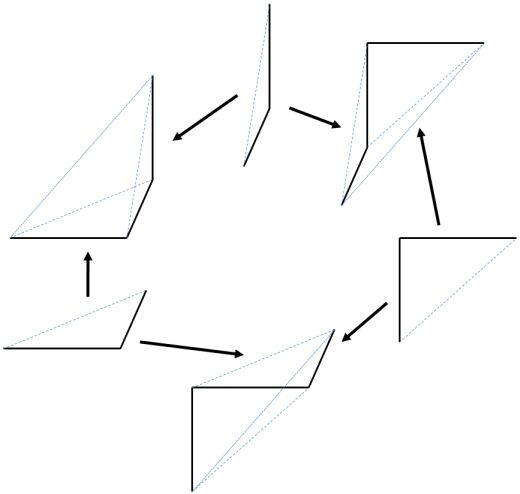Contents

# Contents

## Idea

An interval object $I$ in a category $C$ is an object that behaves in $C$ roughly like the standard topological interval $I \coloneqq [0,1]$ with its two boundary point inclusions

${*}\amalg {*} \stackrel{[0, 1]}{\to} I$

in the category Top of topological spaces, where $[0,1]$ is the copairing of the global elements $0\colon {*} \to I$ and $1\colon {*} \to I$.

A bare interval object may be nothing more than such a diagram. If $C$ admits sufficiently many limits and colimits, then from this alone a lot of structure derives. The precise definition of further structure and property imposed on an interval object varies with the intended context and applications.

Notably in a large class of applications the interval object in $C$ supposed to be the right structure to ensure

1. that there is an object $I$ in $C$ such that for every object $X$ of $C$ the internal hom object $[I,X]$ exists and behaves like a path space object for $X$;

2. that there is a notion of composition on these path objects which induces on $[I,X]$ a structure of a (higher) category internal to $C$: the fundamental category or fundamental groupoid of the object $X$, or rather its fundamental infinity-groupoid.

For instance the choice $C =$ Top and $I = [0,1]$ should be an instance of a category with interval object, and the fundamental algebraic n-groupoid $\Pi_n(X)$ obtained for any topological space $X$ from this data should be the fundamental $n$-groupoid as a Trimble n-category.

We give two very similar definitions that differ only in some extra assumptions.

## Definitions

### Plain definition

###### Definition (plain interval object)

A plain interval object in a category $C$ is just a cospan diagram with equal feet

$pt \stackrel{0}{\to} I \stackrel{1}{\leftarrow} pt$

in $C$, with $I$ and $pt$ any two objects and $0$ and $1$ any two morphisms.

In categories with finite limits it is often required that $pt=*$ is the terminal object and in this case the interval object is called cartesian interval object.

Examples for the use of this notion is at fundamental (infinity,1)-category in the section “fundamental geometric ∞-categories”.

#### Contractible interval object

###### Definition (contractible interval object)

A contractible interval object in a category $C$ with a terminal object $*$ is an object $I$ with two global elements $0:* \to I$ and $1:* \to I$ such that

• $0 = 1$
• for any object $A$ in $C$ and global elements $0_A:* \to A$ and $1_A:* \to A$ such that $0_A = 1_A$, there exists a morphism $f:I \to A$ such that $f \circ 0 = 0_A$ and $f \circ 1 = 1_A$.
###### Definition (contractible interval object in (n, 1)-categories)

A contractible interval object in an $(n, 1)$-category $C$ with a terminal object $*$ is an object $I$ with two global elements $0:* \to I$ and $1:* \to I$ and an equivalence $s:0 \cong 1$ such that

• for any object $A$ in $C$ and global elements $0_A:* \to A$ and $1_A:* \to A$ with an equivalence $s_A:0_A \cong 1_A$, there exists a morphism $f:I \to A$ with a morphism $f^{'}:(0 \cong 1) \to (0_A \cong 1_A)$ such that the equivalences $p:f \circ 0 \cong 0_A$, $q:f \circ 1 \cong 1_A$, and $r:f^{'}(s) \cong s_A$ satisfy the coherence laws.

The universal property of the contractible interval object means that the contractible interval object corresponds to the interval type in Martin-Löf type theory/homotopy type theory, and is thus equivalent to the terminal object. If a cartesian closed $(n,1)$-category has an contractible interval type, the terminal object is a separator (see Mike Shulman’s blogpost).

### In homotopical categories

If the ambient category $C$ is a homotopical category, such as a model category, there are natural further conditions to put on an interval object:

#### Trimble interval object

The following definition is strongly related to the notion of Trimble omega-category where the interval object gives the internal hom $[I,X]$ the structure of an operad giving (by induction) the model of an $A_\infty$-category structure on

$(X_0 := [pt,X]) \stackrel{s := [\sigma,X]}{\leftarrow} [I,X] \stackrel{t := [\tau, X]}{\to} (X_0 := [pt,X]) \,.$

This internal $A_\infty$-category is denoted

$\Pi_1(X)$

This is in (a bit) more detail in Trimble omega-category and in fundamental (infinity,1)-category in the section “Fundamental algebraic $\infty$-categories”.

###### Definition (Trimble interval object)

A category with interval object is

• a symmetric closed monoidal homotopical category $V$;

• with tensor unit being the terminal object, which we write $pt$;

• equipped with a bi-pointed object

$\array{ && I \\ & {}^\sigma \nearrow && \nwarrow^{\tau} \\ pt &&&& pt }$

in $V$, with $I$ called the interval object;

such that

• the pushout

$\array{ && I^{\vee 2} := I \amalg_{pt} I \\ & \nearrow && \nwarrow \\ I &&&& I \\ & {}_{\tau}\nwarrow && \nearrow_{\sigma} \\ && pt }$

exists in $V$, so that all compositions

$\array{ && I^{\vee n} \\ & {}^\sigma \nearrow && \nwarrow^{\tau} \\ pt &&&& pt }$

of $n \in \mathbb{N}$ copies of the co-span $I$ with itself by pushout over adjacent legs exist in $V$;

• and for all $n$, the $V$-objects of morphisms ${}_{pt}[I, I^{\vee n}]_{pt}$ of cospans (as described at co-span) are weakly equivalent to the point

${}_{pt}[I, I^{\vee n}]_{pt} \,.$

#### Berger–Moerdijk segment- and interval object

In section 4 of

the following definition is given:

Let $V$ be a monoidal model category and write $pt$ for the tensor unit in $V$ (not necessarily the terminal object).

A segment (object) $I$ in a monoidal model category $V$ is

• a factorization

$pt \amalg pt \stackrel{[0 , 1]}{\to} I \stackrel{\epsilon}{\to} pt$

of the codiagonal morphism

$pt \amalg pt \stackrel{[Id , Id]}{\to} pt$

from the coproduct of $pt$ with itself that sends each component identically to $pt$.

• together with an associative morphsim

$\vee : I \otimes I \to I$

which has 0 as its neutral and 1 as its absorbing element, and for which $\epsilon$ is a counit.

If $V$ is equipped with the structure of a model category then a segment object is an interval in $V$ if

$[0, 1]\colon pt \amalg pt \to I$

is a cofibration and $\epsilon : I \to pt$ a weak equivalence.

### Interval type

In homotopy type theory the cellular interval can be axiomatized as a higher inductive type. See interval type for more.

## Examples

• In sSet the standard interval object is the 1-simplex $\Delta$.

• In a category of chain complexes the standard interval is the simplicial chain complex $C_\bullet(\Delta)$ on the 1-simplex, see at interval object in chain complexes.

• The cube category is generated from a single interval object.

• The standard interval object in Cat is the 1st oriental $\{0\to 1\}$ (see co-span co-trace)

• For $V = C =$ Top equipped with with the classical model structure on topological spaces, the topological closed interval $I \colon [0,1]$ (with its Euclidean metric topology) with $pt \stackrel{\sigma, \tau}{\to}I$ the maps to 0 and 1, respectively. This is the standard topological interval. This is the case described in detail at Trimble n-category.

• For $V = \omega Cat$ the category of strict omega-categories the first oriental, the 1-globe $I = \{a \to b\}$ is an interval object. In this strict case in fact all hom objects are already equal to the point ${}_{pt}[I, I^{\vee n}]_{pt} = pt$ and

$(X = [pt,X]) \stackrel{s := [\sigma,X]}{\leftarrow} [I,X] \stackrel{t := [\tau, X]}{\to} (X = [pt,X])$

is a strict co-category internal to $\omega$Cat. In this case, for $X$ any $\omega$-category the $A_\infty$-category $\Pi_1(X)$ is just an ordinary category, namely the 1-category obtained from truncation of $X$. Similarly, probably $\Pi_\omega(X) = X$ in this case.

### Standard intervals, cubes and simplices in $Top$ and $Diff$

Let $X =$ Top or $C =$ Diff be the category of topological spaces or of manifolds.

A standard choice of interval object in $C$ is $I = [0,1] \subset \mathbb{R}$ with the obvious two boundary inclusions $0,1 : {*} \to [0,1]$.

But another possible choice is to let $I = \mathbb{R}$ be the whole real line, but still equipped with the two maps $0,1 : {*} \to \mathbb{R}$, that hit the $0 \in \mathbb{R}$ and $1 \in \mathbb{R}$, respectively.

Either of these two examples will do in the following discussion. The second choice is to be thought of as obtained from the first choice by adding “infinitely wide collars” at both boundaries of $[0,1]$. While ${*} \stackrel{0}{\to}[0,1] \stackrel{1}{\leftarrow} {*}$ may seem like a more natural choice for a representative of the idea of the “standard interval”, the choice ${*} \stackrel{0}{\to} \mathbb{R} \stackrel{1}{\leftarrow} {*}$ is actually more useful for many abstract nonsense constructions.

But since it is hard to draw the full real line, in the following we depict the situation for the choice $I = [0,1]$.

Then for low $n \in \mathbb{N}$ the above construction yields this

• $n=0$ – here $\Delta_I^0 = I^{\times 0} = {*}$ is the point.

• $n=1$ – here $\Delta_I^1 = I^{\times 1} = I$ is just the interval itself

$\array{ (0) \to (1) }$

The two face maps $\delta_1 {*} \to I$ and $\delta_0 : {*} \to I$ pick the boundary points in the obvious way. The unique degeneracy map $\sigma_0 : I \to {*}$ maps all points of the interval to the single point of the point.

• $n=2$ – here $\Delta_i^2 = I^{\times 2} = I \times I$ is the standard square

$\array{ (0,1) &\to& (1,1) \\ \uparrow && \uparrow \\ (0,0) &\to& (1,0) }$

But the three face maps $\delta_i : I \to I\times I$ of the cosimplicial object $\Delta_I$ constructed above don’t regard the full square here, but just a triangle sitting inside it, in that pictorially they identify $(\Delta_I^1 = I)$-shaped boundaries in $I \times I$ as follows:

$\array{ (0,1) &\to& (1,1) \\ \uparrow &^{= \delta_1(I)}\nearrow& \uparrow^{ = \delta_0(I)} \\ (0,0) &\stackrel{= \delta_2(I)}{\to}& (1,0) }$

(here the arrows do not depict morphisms, but the standard topological interval, i don’t know how to typeset just lines without arrow heads in this fashion!)

• $n=3$ – here $\Delta_i^3 = I^{\times 3} = I \times I \times I$ is the standard cube

###### Exercise

Insert the analog of the above discussion here and upload a nice graphics that shows the standard cube and how the cosimplicial object $\Delta_I$ picks a solid tetrahedron inside it.

As a start, we can illustrate how there are 6 3-simplices sitting inside each 3-cube.Once you see how the 3-simplices sit inside the 3-cube, the facemaps can be illustrated as follows:Note that these face maps are to be thought of as maps into 3-simplices sitting inside a 3-cube.

## Fundamental $\infty$-categories induced from intervals

The interest in interval objects is that various further structures of interest may be built up from them. In particular, since picking an interval object $I$ is like picking a notion of path, in a category with interval object there is, under mild assumptions, for each object $X$ an infinity-category $\Pi_I(X)$ – the fundamental $\infty$-category of $X$ with respect to $I$ – whose k-morphisms are $k$-fold $I$-paths in $X$.

This is described for two models for $(\infty,1)$-categories at fundamental (infinity,1)-category

## Homotopy localization induced from an interval

Given a suitable interval obect in a site $C$, one may ask for ∞-stacks on $C$ that are invariant under the notion of homotopy induced by $I$. These are obtained by homotopy localization of a full (∞,1)-category of (∞,1)-sheaves on $C$.

• Clemens Berger, Ieke Moerdijk, The Boardman-Vogt resolution of operads in monoidal model categories (arXiv), section 4, p.11